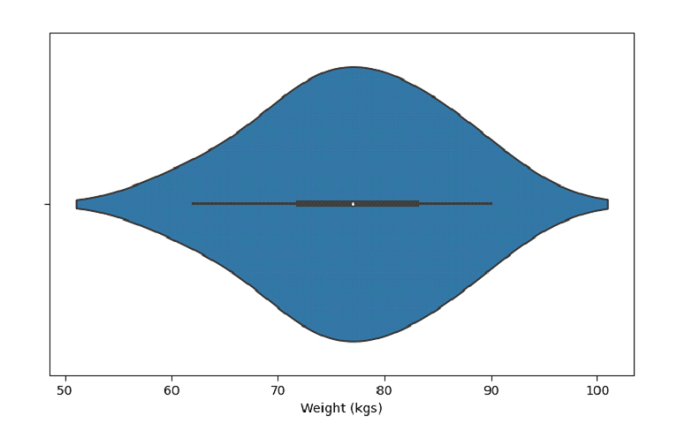# Create a Violin Plot with SeaBorn – Python Pandas

Violin Plot in Seaborn is used to draw a combination of boxplot and kernel density estimate. The seaborn.violinplot() is used for this.

Let’s say the following is our dataset in the form of a CSV file − Cricketers.csv

At first, import the required 3 libraries −

import seaborn as sb
import pandas as pd
import matplotlib.pyplot as plt

Load data from a CSV file into a Pandas DataFrame −

dataFrame = pd.read_csv("C:\Users\amit_\Desktop\Cricketers.csv")


Plotting violin plot with column Weight (kgs) −

sb.violinplot(dataFrame['Weight'])

## Example

Let us see an example −

import seaborn as sb
import pandas as pd
import matplotlib.pyplot as plt

# Load data from a CSV file into a Pandas DataFrame:

# plotting violin plot with Weight (kgs)
sb.violinplot(dataFrame['Weight'])

# set label
plt.xlabel("Weight (kgs)")

# display
plt.show()

## Output

This will produce the following output −